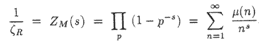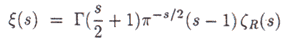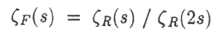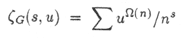## "Statistical theory of numbers"

Bernard L. Julia

Laboratoire de physique Theorique de l'Ecole Superieure, 24, Rue Lhomond, F-75231 Paris Cedex 05, France

[from Number Theory and Physics, Springer Proceedings in Physics, Vol. 47, Editors:J.M. Luck, P. Moussa, M. Waldschmidt (Springer-Verlag Berlin, Heidelberg 1990) pp. 276-293]

Abstract. This article is three-sided: it is a pedestrian introduction to the Riemann hypothesis in the case of the classical zeta function, it provides also an elementary presentation of Quantum statistical Mechanics and finally it is an attempt to present the reader with a precise dictionary between the fields of number theory - additive or multiplicative - on the one hand and of thermodynamics of "solved quantum systems" on the other, the physical terms mentioned in the mathematical part will be defined in the Physics section.

### 1. Introduction

In his famous memoir of 1859  Riemann started the field of analytical number theory and stimulated the development of a good fraction of modern Mathematics. At that time Boltzmann was only 15 years old, he was to derive together with Gibbs the results of thermodynamics from the simpler principles of Statistical Mechanics. Let us take note that 1859 is also the date of the first communication by Maxwell on the dynamical theory of gases. After Planck Quantum Statistical Mechanics was developed in parallel with Quantum mechanics, the Bose-Einstein and Fermi-Dirac statistics date back respectively to 1924 and 1926.

In 1989 we are accustomed to the occurrence of theta functions and modular forms in the quantum theory of strings and in the partition functions of statistical mechanics on a two-dimensional torus. These expressions are related to generating functions of additive number theory. Here we would like to argue that multiplicative number theory which is best encoded through Dirichlet series corresponds also to some physical systems; we do not know yet their Hamiltonian form but we know their spectrum and we can discuss their thermodynamics.

As one may expect the primes play the role of partons and their quantum "statistics" is at our disposal, different choices lead to distinct classical series. This is one meaning of the word statistics. Another one arises: the "statistical" distribution of the primes on the real line is also connected with the Riemann hypothesis. The title of this article has been chosen despite this ambiguity. A most interesting connection seems however the fact that one can reinterpret the pole of the zeta function at s = 1 as a breakdown of the grand-canonical ensemble at the Hagedorn  temperature because of the exponential increase of the density of energy levels for large energies. Lee-Yang type theorems restrict the locus of the zeros of partition functions that may be related to phase transitions. The Riemann hypothesis is similar. Here we shall meet zeros and also poles in solved systems. The thermodynamic formalism leads us to generalize various generating functions of number theory and may help in their study.

[Commentary/summary]

### 2. Some generating functionals of number theory

2.1 The Zeta function of Riemann

Julia introduces the zeta function zetaR and shows that both it and its inverse ZM = 1/zetaR are examples of Dirichlet series. The analytic continuation and functional equation of the zeta function are discussed. The Euler product representation is given, and it is deduced thatWhere mu counts the parity of the number of distinct prime factors of n and vanishes whenever n is not "squarefree" (i.e. when n is divisible by a square).

Dirichlet L functions and other generalisations of the zeta function are then briefly described.

2.2 Equivalent forms of the (classical) Riemann hypothesis

Four forms are given:

• The function xi(s) vanishes only on the axis Re(s) = 1/2, where• The abscissa of convergence of ZM is equal to 1/2.
• The Mertens function M(x) does not grow faster than x1/2+e for any positive e, where M(x) = {Sum n less than x} mu(n
• (pi(x) - Li(x))/Li(x) is less than xe-1/2 for any positive e and sufficiently large x, where pi(x) is the prime counting function, and Li the logarithmic integral Li(x) = {Integral from 2 to x} 1/log y dy.
2.3 Generating functionals

2.4 Möbius and Mellin transforms

### 3. Quantum Statistical Mechanics

3.1 Basic tools of QSM

3.2 QSM without Hamiltonian

3.3 Zeros and poles of partition functions

"Putting together the concepts of the last section and those of part 2, we are led to look for equalities between partition functions and generating functions. The inspiration may come from the Möbius function which strongly suggests a fermionic character for the prime numbers as does the concept of squarefree numbers. The key remark is now that energy is the additive quantity of thermodynamics, in multiplicative number theory the additive quantities are the logarithms of the integers, so let us identify the two theories.

A particle physicist naturally wants to break numbers into partons: they will have energies log p for the prime numbers. The energy levels will depend on the statistics of these primes. In the fermionic case they will be the logarithms of squarefree numbers but if we insist on treating them as bosons they will correspond to the (multiplicative) nonzero integers. The unique factorization of integers ensures that each nonzero integer appears exactly once. Now the number of factors may or may not be fixed. It turns out that mathematicians have encountered the second (i.e. grand-canonical) situation which is usually easier to handle in Physics also. Let us call the bosonic case the free Riemann gas because its grand-canonical partition function at zero mu (fugacity) is nothing but the Riemann zeta function. Its inverse is not quite a partition function because of the minus signs. It is the supertrace of the operator e-sH. The proper treatment of the fermionic case leads to the generating series of |mu(n)|:.Let us remark that our choice involves some arbitrariness but it seems the most natural. The same idea allows us to reinterpret several classical objects of mathematics. ZM (= 1/zeta) can be interpreted as a partition function of particles with indefinite metric, the primes are then "ghost" states, let us call this system the Möbius gas.

It is obvious now to interpret the Möbius transform as the tensor product of a model by the Riemann gas, and its inverse as the tensoring by the Möbius gas."

### 4. Conclusion

We are left with a dictionary and a program. One of the natural questions to ask is whether the introduction of a chemical potential that is the replacement of the zeta function of two variables s and u helps to avoid the pole at s = 1. The classical example of Bose condensation shows that one must allow non-vanishing chemical potentials above the critical temperature of decondensation to find the equilibrium distribution, but in the case of fugacity u = -1 the Dirichlet series is well known and it is not known to converge better that zetaR. We shall return in the announced mathematical article to the formulation of a generalized Riemann hypothesis for the functionWhere Omega(n) is the number of prime factors (distinct or not) of n. The case of Dirichlet L functions is also interesting because of the absence of pole.

Let us apologize to the many people who have made one or all of these trivial remarks before and are not quoted here. We keep discovering precursors of what we once thought was original but rather formal work. Some dates are given in . Mackey discussed the bosonic case but did not really introduce fermions. Several other approaches ought to be related to the present one: the work of M. Berry and others on dynamical systems with closed orbits of length log p and the Selberg zeta function, the detailed study of zeros of partition functions and their concentration on sets of low dimensionality, etc

### References

 B. Riemann, Monatberichte der Berliner Akad. (1859) November.

 R. Hagedorn, Suppl. Nuovo Cimento 3 (1965) 147. See also Yu. B. Rumer, J. Exp. Theor. Phys 38 (1960) 1899.

 J.P. Serre, Cours d'Arithmetique PUF, Paris 1977.

 A. Weil, Collected papers, Vol. I, 399-410

 G.H. Hardy and E.M. Wright. An introduction to the theory of numbers, Clarendon Press, Oxford 1945.

 H.M. Edwards, Riemann's zeta function, Academic Press, New York 1974.

 K. Huang, Statistical Mechanics, Wiley, New York 1963

 R.J. Baxter, Exactly solved models in Statistical Mechanics, Academic Press, New York 1982

 M.E. Fisher in W.E. Brittin, Boulder Lectures in Theoretical Physics 1964, University Of Colorado Press, Boulder 1965.

 B.L. Julia, in preparation

 B.L. Julia 1985 unpublished; B.L Julia, Journal de Physique 50 (1989) 1371; D. Spector, Utrecht preprint THU '88/41; J. Hannay, unpublished according to M. Berry; G.W. Mackey, Unitary Group Representation in Physics, Probability and Number Theory, Benjamin, Reading 1978; Unknown

archive      tutorial      mystery      new      search      home      contact